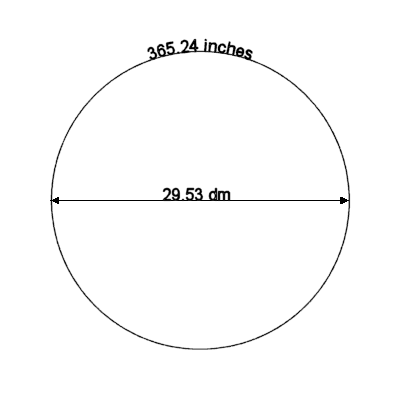## Relationships Between Decimeter, Inch, Year, and MonthWhat do inches and decimeters (1 decimeter = 10 centimeters) have to do with astronomy? That's what first popped into my mind when I discovered this amazing relationship. Suppose you have a circle with a diameter measured in decimeters and a magnitude equal to exactly 1 synodic month. We'll use the value of 29.53 for the length of the synodic month. It turns out that the magnitude of the circumference of the circle, measured in inches matches the length of the tropical year in days.

Math:
One year = 365.24 days
One synodic month = 29.53 days
29.53 days × π × 1 dm/day = 92.771 dm
92.771 dm ÷ 0.254 dm/inch × 1 day/inch= 365.24 days

In fact, this calculation is so accurate that if you made a disk to these dimensions, you would have to have a tolerance of finer than 1/10 millimeter before you'd be able to discern the error. The calculation of this error is left as an exercise for the reader.